International Journal of Applied Mathematics and Theoretical Physics
Volume 2, Issue 4, October 2016, Pages: 28-30

On the Perturbation Theory in Quantum Electrodynamics Using the Wave Functions of the Dressed States

Yuriy N. Zayko

Department of Applied Informatics, Faculty of Public Administration, Russian Presidential Academy of National Economy and Public Administration, Stolypin Volga Region Institute, Saratov, Russia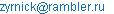Yuriy N. Zayko. On the Perturbation Theory in Quantum Electrodynamics Using the Wave Functions of the Dressed States. International Journal of Applied Mathematics and Theoretical Physics. Vol. 2, No. 4, 2016, pp. 28-30. doi: 10.11648/j.ijamtp.20160204.11

Received: August 4, 2016; Accepted: August 15, 2016; Published: September 6, 2016

Abstract: The paper considers the possibility of constructing a perturbation theory for problems of quantum electrodynamics, which is based on the wave functions of so-called "dressed" electron, unlike traditional perturbation theory, which uses the wave functions of the "bare" electrons. To investigate the wave functions of the "dressed" electron a numerical investigation of associated Dirac-Maxwell equations was performed in the approximation of small electron pulses. An expression for the energy eigenvalues of the considered self-consistent problem was found in a quasi-classical approximation as well as an estimation of the lifetime of the "dressed" electron and the effective value of the electron charge.

Keywords: Photon, Electron, Quantum Electrodynamics, Perturbation Theory, Divergence, Renormalization

Contents

1. Introduction

Modern quantum field theory (QFT), without a doubt, is the most rapidly developing branch of theoretical physics. Its results are used in many related areas: the theory of elementary particles, cosmology, condensed matter physics, etc. All of the results of field theory can be divided into two classes, obtained in the framework of perturbation theory (perturbative) and the so-called non-perturbative. The first class includes, for example, the results obtained using the method of S - matrix. The boundary of the second class are more difficult to delineate because it is constantly expanding, adding new results. More details on this matter could be found for example in books [1,2].

In recent years, the development of QFT is marked by the rapid development of the second trend, as the most interesting and promising. Results of the first direction are limited, mainly by the search of renormalization methods in various models of QFT aimed at the elimination of non-physical divergences in expressions for observable quantities. It is clear that the divergences - are the inevitable defect of perturbation theory, which uses as functions of zero approximation the wave functions of the "bare" particles that do not exist in nature 1.

It is possible to correct this deficiency of theory of perturbation, choosing as the functions of zero approximation wave functions of "dressed" particles. For example, in quantum electrodynamics, such functions correspond to states of particles surrounded by a cloud of virtual photons. The present work is devoted to the study of such states.

2. Investigation of Maxwell-Dirac Equations

At the heart of quantum electrodynamics (QED) lays the Dirac equation for a particle of spin ½ which are solved together with Maxwell's equations. We investigate these equations using well-known expansion of the Dirac equation in powers of с-1, where с – is a speed of light in vacuum . In this approximation, the two components of the Dirac bi-spinor corresponding to the positron states are small, and the equation for the two other (electronic) components, denoted φ, are connected with Maxwell's equations, which are reduced to equation for the electric field, where Φ- is a scalar potential. The final equations look as follows :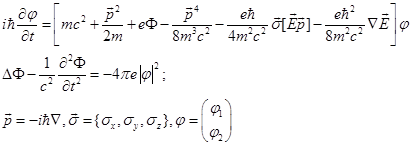(1)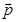- the electron pulse operator,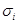- the Pauli matrices,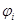- spinor component corresponding to the opposite directions of spin (i =1, 2), е – elementary electric charge, m –mass of an electron. Equations (1) are valid with the precision up to с-2 . If we additionally put a requirement of smallness of the electron velocity, in the first equation can be omitted amendments ~ c-2 containing operator. We also assume spherical symmetry of the problem. In the static case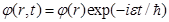and introducing value, we reduce the equations (1) to the system of equations(2)

Term ~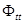in the second equation (1) is connected with the photon delay. It was omitted on the assumption that the size of the region of interaction Δr of photons and electrons is small and the delay effect ~ (Δr/c)2 can be neglected. In this regard, it is also logical to drop the term ~c-2 in the first equation because its taking into account leads to the excess accuracy. Then the system (2) consists with the Schrödinger equation for the electron whose spin isn't taken into account, and the equation for the electric field which is created by electron itself. Member ~c-2only introduces additional nonlinearity.

It is convenient to introduce the variable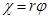, equations for which look as follows: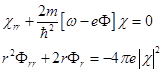(3)

This system should be supplemented by the boundary conditions, which we choose as the conditions that electron wave function at infinity (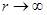) contains only outgoing wave.

Let us rewrite the equation (3) in dimensionless form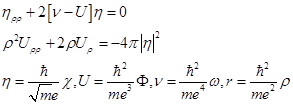(4)

Recall that due to the spinor nature of η the first equation (4) represents two equations for each componentregardless to their entanglement, and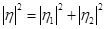.

If we change the sign of the right side of the second equation to the opposite one, then we arrive at the well-known system of Schrödinger-Newton equations which were numerically investigated in the works [6,7]. By analogy, the equations (4) can be called as Schrödinger-Maxwell equations, or given made simplifications as the Schrödinger-Poisson equations. With their help, we can explore the behavior of an electron being in a cloud of virtual photons.

3. Numerical Study of the Equations (4)

Fig. 1 shows the results of a numerical study of the equations (4)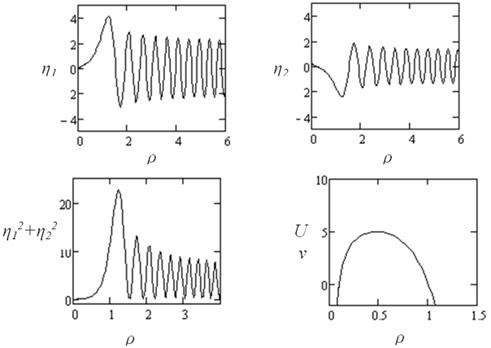Fig. 1. The results of the numerical study of the equations (4) for ν = 0, i.e. the energy is measured from this level. Initial values are set in the left turning point: η1 = η2 = 0.1, η1΄ = - η2 ΄= 0.1, U = 0, U΄ = 70.

The results show that the electron which is surrounded by a cloud of virtual photons ("dressed" electron) is in a quasi-stationary state, which is separated from the free state ("bare") electron by potential barrier. Since the value η2near ρ = 0 varies slowly, the second equation in (4) can be integrated what leads to(5)

Z and В are constants, defined by the boundary conditions. Due to smallness of η2(0)≈0,02 the behavior of the potential U is defined by second two terms in (5). For small ρ the main contribution to the potential gives the Coulomb term, i.e., U(ρ ~ 0) ≈Zρ-1, Z has a sense of the real charge of the electron, shielded by cloud of virtual photons. Make it easier for all by plotting U(ρ-1)(Fig. 2). Value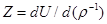, that can be numerically estimated for the selected boundary conditions approximately as Z ≈ -0,6 (in the units of bare electron charge) and doesn’t vary in the wide range of energy. For the same data В ≈ 7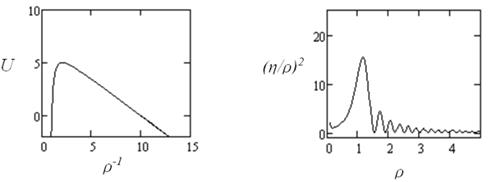Fig. 2. Left: the dependence of U(ρ-1). Liner section (large ρ-1) corresponds to the screened Coulomb interaction at small distances. Right: the screening charge density (η/ρ)2.

If we measure the energy of the electron from the value of U at its maximum, then the expression for the energy levels in the quasi-classical approximation looks as follows :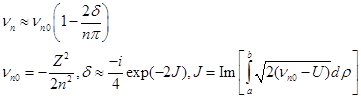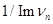(6)

where n = 1,2,…- is a set of integers bounded above, J – imaginary action received under the barrier, a and b are the turning points of the integrand for J >> 1. To receive (6) we used formulas of connection for quasi-classical solutions on either side of the potential barrier and the Bohr-Sommerfeld condition for finding the energy levels .

In other words, the electron tunneling under the barrier can with small but finite probability to get rid of the cloud of photons and become "bare". Life time of "dressed" electron Δt can be evaluated with the help of the Heisenberg condition of indeterminacy Δt ≥ ћ/Δε, where ε = εn = Re(ћωn) is the energy of dressed state and Δε = Im(ћωn) is a width of the state. So the lower bound of the life time may be estimated as. The nature of the resulting expression corresponds to the resonant structure of the scattering processes involving electrons and photons. The number of these resonances are determined by the number of quasi-stationary states-solutions of the Schrödinger equation in the potential U.

4. Conclusion

In this paper we attempt to address some problems of field theory without the use of traditional perturbation theory using as an unperturbed states the free states of particles whose interaction is described in the highest orders of the theory.

In the problem of electron-photon scattering, the state of "dressed" electron was described and the likelihood of its transformation to a "naked", i.e. without the cloud of virtual photons was evaluated.

Since at given approach the interaction of electrons with photons is not assumed as point-like, calculating the screened charge doesn't lead to a problem inherent to standard theory of perturbation . However, should not be given much importance to the calculated value of Z because for other boundary conditions, it can be changed.

To give more credibility to the results one should justify the choice of the set of boundary conditions for the equations (4), which is rather complicated independent computational problem that is not solved yet. We only say that the variation of these boundary conditions in the wide enough vicinity of the selected ones leads to a minor quantitative changes in the character of the solution.

The using of "dressed" states in QED seems useful and perspective in many purposes. In  this states (so called "Volkov states") are used for the calculation of high order harmonic generation for hydrogen-like atom in the field of ultrashort intense laser pulse.

Searching of new methods in the theoretical calculations in QED is needed because accuracy of experimental measurements very prevail in comparison with theoretical possibilities, what is outlined in a number of works [10,11,12].

References

1. Rajaraman R. An Introduction to Solitons and Instantons in Quantum Field Theory. North-Holland Publishing Company, Amsterdam-NY-Oxford (1982).
2. Zee A., Quantum Field Theory in a Nutshell. 2nd ed. Princeton University Press, 2007.
3. Feinberg E.L. Uspekhi Fizicheskih Nauk, V. 132, № 2, pp. 255-291, 1980 (Russian).
4. Berestetskii V.B., Lifshitz E.M., Pitaevskii L.P., (1971). Relativistic Quantum Theory. Vol. 4 (1st ed.). Pergamon Press.
5. Bohm D., Quantum Theory. New York: Prentice Hall; 1989
6. Harrison R, Moroz I. and Tod K. P.. A numerical study of the Schrȍdinger–Newton equations, Nonlinearity V. 16, p.101–122, 2003.
7. Moroz M., Penrose R. and Tod P. Spherically-symmetric solutions of the Schrȍdinger–Newton equations, Class. Quantum Grav. V. 15 2733–2742, 1998.
8. Landau L.D., Pomeranchuk I.Ya. On the point-like interaction in quantum electrodynamics. Doklady Akademii Nauk USSR, (1955), V. 102, p. 489.(Russian).
9. Hu H. and Yuan J. Non-perturbative QED Model with Dressed States to Tackle HHG in Ultrashort Intense Laser Pulses.- Phys. Rev. A 78, 063826, 2008.
10. Lindgren I., Salomonson S. and Holmberg J. Energy-dependent perturbation theory: Possibility for improved tests of quantum-electrodynamics.- arXiv:1212.6482, (28.12.2012)
11. Neznamov V.P. Anomalous magnetic moment and Lamb shift in the second order of the perturbation theory with finite electron mass renormalization in QED. arXiv:hep-th/0403125v2, (20.04.2004).
12. Jentschura U. D. Quantum Electrodynamic Bound-State Calculations and Large-Order Perturbation Theory. arXiv:hep-ph/0306153, (27.04.2004).

Footnotes

 The possibility of "bare" particles detection in the scattering experiments was often mentioned by E.L. Feinberg (see, for example, )

 Contents 1. 2. 3. 4.
Article ToolsAbstractPDF(332K)